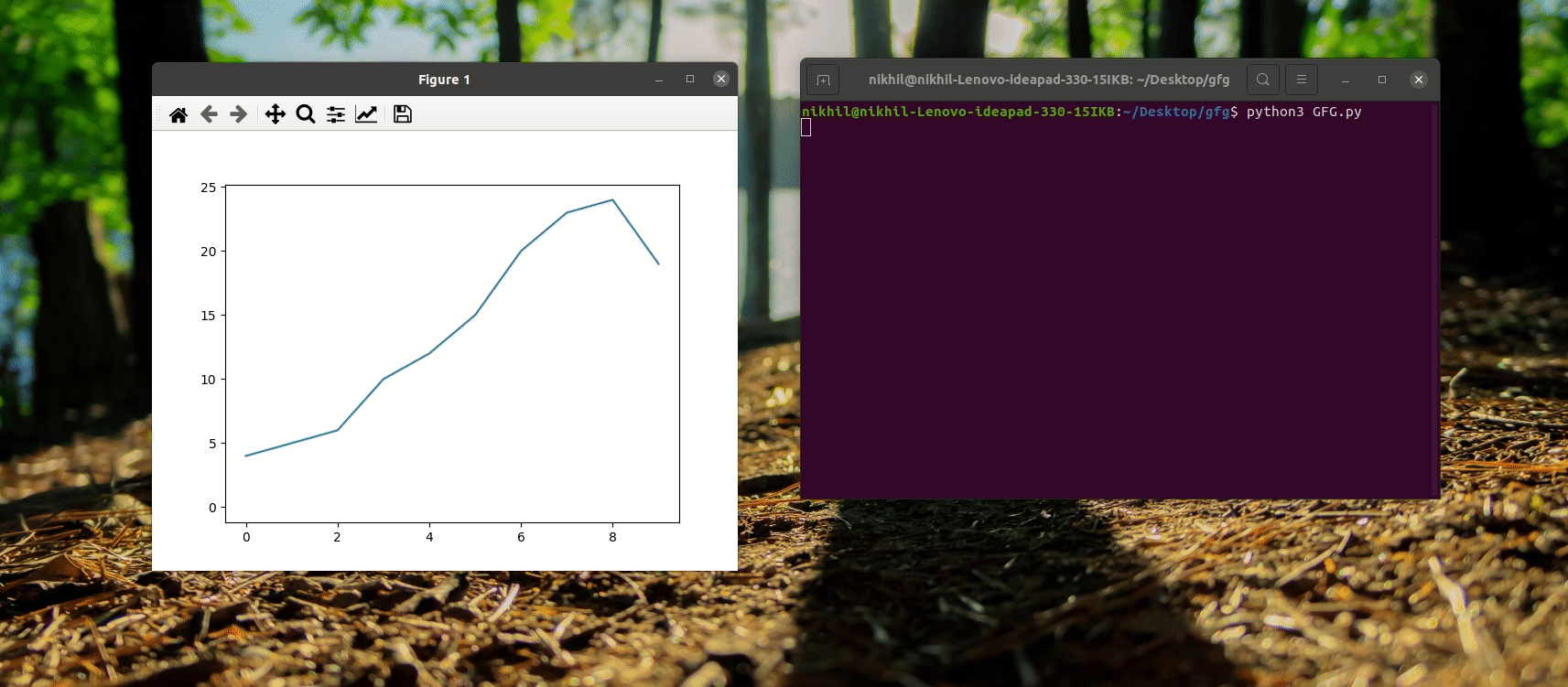Related Articles
Matplotlib – Rectangle Selector
• Last Updated : 23 Feb, 2021

Matplotlib is a python library for visualization. It provides various widgets to make the visualization of data simple. There are some cases when there is a need of selection of particular region of a graph. For this interactivity, Matplotlib provides RectangleSelector widget. This widget helps to select a rectangular region of given axes. Also, it provides a way to perform actions according to the selection.

RectangleSelector(): Selects a rectangular region of given axes.

Syntax: class matplotlib.widgets.RectangleSelector(ax, onselect, drawtype=’box’, minspanx=0, minspany=0, useblit=False, lineprops=None, rectprops=None, spancoords=’data’, button=None, maxdist=10, marker_props=None, interactive=False, state_modifier_keys=None)

Parameters:

• ax: A matplotlib.axes.Axes instance where widget is placed.
• onselect: Function to connect to the selection event. When the selection is completed, respective function is called. onselect function takes mouse click and mouse release events as arguments.
• drawtype: It specifies how to show selection. If “box”, then draws the full rectangle box. If “line”, then draws line of rectangle. If “none”, then draws nothing.  Default value is “box”.
• button:  It provides list of mouse buttons that can trigger rectangle selection. By deafult all buttons are allowed.
• maxdist: It is a distance in pixels within which the interactive tool handles can be activated. Default value is 10.
• interactive: It is a boolean value to specify whether to draw a set of handles for interaction with the widget.

Properties:

• center: Provides center of rectangle drawn
• corners: Provides corners of rectangle starting from lower left, moving clockwise.
• edge_centers: Provides midpoint of rectangle edges starting from left moving clockwise.
• extents: Returns (xmin, xmax, ymin, ymax).
• geometry: Returns an array containing the x and y co-ordinates of the four corners of the rectangle (starting and ending in the top left corner). Array shape is (2, 5).  all x coordinates can be obtained using RectangleSelector.geometry[1, :] and y coordinates using RectangleSelector.geometry[0, :].

Methods:

• draw_shape(self, extents): draws a rectangular shape using (xmin, xmax, ymin, ymax) values.

Example 1:

Following program demonstrates simple RectangleSelector used to select a region and zoom the selected area.

## Python3

 `from` `matplotlib.widgets ``import` `RectangleSelector``import` `matplotlib.pyplot as plt`` ` `# Function to be executed after selection``def` `onselect_function(eclick, erelease):``   ` `    ``# Obtain (xmin, xmax, ymin, ymax) values``    ``# for rectangle selector box using extent attribute.``    ``extent ``=` `rect_selector.extents``    ``print``(``"Extents: "``, extent)`` ` `    ``# Zoom the selected part``    ``# Set xlim range for plot as xmin to xmax``    ``# of recatngle selector box.``    ``plt.xlim(extent[``0``], extent[``1``])``     ` `    ``# Set ylim range for plot as ymin to ymax``    ``# of recatngle selector box.``    ``plt.ylim(extent[``2``], extent[``3``])`` ` `# plot a line graph for data n``fig, ax ``=` `plt.subplots()``n ``=` `[``4``, ``5``, ``6``, ``10``, ``12``, ``15``, ``20``, ``23``, ``24``, ``19``]``ax.plot(n)`` ` `# Define a RectangleSelector at given axes ax.``# It calls a function named 'onselect_function'``# when the selection is completed.``# Rectangular box is drawn to show the selected region.``# Only left mouse button is allowed for doing selection.``rect_selector ``=` `RectangleSelector(``    ``ax, onselect_function, drawtype``=``'box'``, button``=``[``1``])`` ` `# Display graph``plt.show()`

Output:Attention geek! Strengthen your foundations with the Python Programming Foundation Course and learn the basics.

To begin with, your interview preparations Enhance your Data Structures concepts with the Python DS Course.

My Personal Notes arrow_drop_up